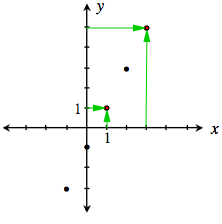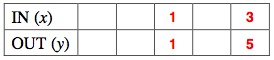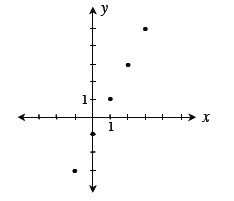### Home > AC > Chapter 3 > Lesson 3.2.2 > Problem3-86

3-86.

Examine the graph at right.

1. Use the graph to complete the table:

 IN ($x$) OUT ($y$)1. Use the graph to find the rule:
$y=$ _____

The graph is a line, so the equation must be the equation of a line.